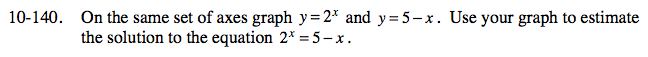### Home > CCA > Chapter 10 > Lesson 10.3.3 > Problem10-140

10-140.

On the same set of axes graph y = 2x and y = 5 − x. Use your graph to estimate the solution to the equation 2x = 5 − x. 10-140 HW eTool (Desmos). Homework Help ✎Use the same method as problem 10-130 from Lesson 10.3.2 to find the solution.

Type the equations into boxes 3 and 4 below. Then estimate the solution.
Click on the link at right for the full eTool version: CCA 10-140 HW eTool